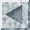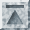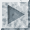# Descriptive Statistics

SST will compute a wide variety of descriptive statistics on your data set. These include means, standard deviations, ranges, correlations, one-way and multi-way cross-tabulations. These procedures are useful for checking data entry and exploring simple relationships between variables.

## Setting the range for procedures

Each SST statistical procedure allows you to restrict the range of observations over which statistics will be computed using the `IF` and `OBS` subops. However, you may want to use the same range for several procedures and avoid typing the `IF` and `OBS` subops over and over. To do this, issue a `RANGE` statement with the `IF` or `OBS` subops. For example:

```range obs[100-200] if[x > 0]
```

Until you issue a new `RANGE` statement, only observations numbered between 100 and 200 for which the value of the variable `x` is positive would be used.

## Obtaining frequency distributions

The `FREQ` command calculates one-way frequency distributions. In our example, the command:

```freq var[party]
```

produces the following output:

```    party

0           1
Democrat       Repub
----------  ----------
Count              12           9
Percent         57.14       42.86
```

Across the top of the frequency table are the values of the variable `party`. Underneath each value is its label (if any). The rows of the table give the number of observations taking the value and the percent of nonmissing observations taking that value.

## Contingency tables

The `TABLE` command is used to produce two-way, three-way, and higher dimensional contingency tables. `TABLE` crosstabulates the first variable specified in the `VAR` subop (the row variable) by the second variable in the `VAR` subop (the column variable). In its simplest and most common use, only two variables will be specified and one table will be created.

Output from the `TABLE` command shows the number of occurrences in each cell as well as the percentage this represents of the column. If one wants percentages of the row total instead, specify the `ROW` subop.

Contingency tables are most useful when the data for each variable only takes on only a few values. In our example, most of the variables are continuous (taking many distinct values, with the same value rarely occuring more than once) so a contingency table on these variables directly would be of little use. One possibility is to recode these variables into a few categories. For our purposes, two categories will be enough for each variable:

```recode var[money inflat unemp] map[(lo thru 70=0,else=1]
```

We will label the categories `low` and `high`. First we try a two-way table:

```table var[inflat money]
```

The `TABLE` output is:

```********** Crosstabulation of inflat by money **********

money
|---------|---------|---------|
|  COUNT  |      low|     high|   ROW
| COL PCT |        0|        1|  TOTAL
inflat|---------|---------|---------|
|        0|     13  |      3  |     16
|      low|   81.3  |   60.0  |   76.2
|---------|---------|---------|
|        1|      3  |      2  |      5
|     high|   18.8  |   40.0  |   23.8
|---------|---------|---------|
COLUMN       16         5        21
TOTAL     76.2      23.8     100.0
```

Note that there are 13 observations for which both money and inflat are zero, which means that both money growth and inflation are under seven percent. The numbers around the edges of the table are row and column marginal totals. For example, the number of `low` values for the variable `money` is given by the column total 16. Similarly for the row totals give the marginal distribution of the row variable `inflat`. The 16 observations for which inflation is `low` (i.e., under seven percent) represent 76.2% of the total of 21 observations.

The default is for SST to compute column percentages. If you would like for the cell entries to be row percentages, specify the subop `ROW` and SST will calculate percentages this way.

SST will also compute chi-square statistics for testing the independence of two discrete variables if you add the `MEASURES` subop to the `TABLE` command:

```table var[money inflat] measures
```

SST prints out the value of the chi-square statistic for the table along with its degrees of freedom. The hypothesis of independence is rejected if the computed value of this statistic exceeds the critical value for corresponding to the significance level chosen for the test. The critical value is chosen so that the probability of obtaining a value of the test statistic larger than the critical level if the null hypothesis of independence is correct is equal to the significance level. The critical value can be determined by consulting a table of the chi-square distribution.

Instead of determining the critical value from a table of the chi-square distribution, you may prefer to compute a p-value for the test statistic. The p-value is the probability (under the null hypothesis of independence) of obtaining a value of the test statistic greater than or equal to the observed value of the test statistic. Suppose for example that SST produces a chi-square statistic of 5.02 with one degree of freedom. The `CALC` command can be used to evaluate the upper tail probability:

```calc 1.0-cumchi(5.02,1)
0.025
```

Thus the p-value for this test statistic is 0.025. This means that if the significance level for the hypothesis test is larger than 0.05, then the null hypothesis can be rejected.

## Multiway contingency tables

Finally, n-way tables can be produced by specifying additional variables in the `VAR` subop. For example:

```table var[money inflat unemp]
```

will produce two tables. The variable `unemp` has been recoded so that it takes two values (zero and one). The `TABLE` command will produce a cross-tabulation of the variables `money` and `inflat` for each value of the variable `unemp`:

```********** Crosstabulation of money by inflat **********

unemp = low ( 0 )

inflat
|---------|---------|---------|
|  COUNT  |      low|     high|   ROW
| COL PCT |        0|        1|  TOTAL
money|---------|---------|---------|
|        0|     12  |      1  |     13
|      low|   85.7  |   33.3  |   76.5
|---------|---------|---------|
|        1|      2  |      2  |      4
|     high|   14.3  |   66.7  |   23.5
|---------|---------|---------|
COLUMN       14         3        17
TOTAL     82.4      17.6     100.0

********** Crosstabulation of money by inflat **********

unemp = hi ( 1 )

inflat
|---------|---------|---------|
|  COUNT  |      low|     high|   ROW
| COL PCT |        0|        1|  TOTAL
money|---------|---------|---------|
|        0|      1  |      2  |      3
|      low|   50.0  |  100.0  |   75.0
|---------|---------|---------|
|        1|      1  |      0  |      1
|     high|   50.0  |    0.0  |   25.0
|---------|---------|---------|
COLUMN        2         2         4
TOTAL     50.0      50.0     100.0
```

If additional variables are specified in the `VAR` subop, a separate table crosstabulating the first and the second variables for each combination of values in the remaining variables will be created. In this manner, an n-way table is constructed.

## Univariate statistics

The `COVA` command computes descriptive statistics (means, standard deviation, ranges, correlations) on a set of one or more variables. We will first consider the use of the `COVA` command for producing univariate statistics. If you do not include any subops other than the `VAR` subop, `COVA` will calculate by default the mean, minimum, maximum, and standard deviation of each variable in the `VAR` subop. Using an asterisk (`*') to match all variables, we obtain a complete set of univariate statistics for the variables in memory:

```cova var[*]
```

SST produces the following output:

```          nobs        mean         min         max     std dev
year        21        1970        1960        1980       6.055
money       21         5.3         0.7         9.3       2.211
inflat      21       4.729         0.9         9.3       2.625
unemploy    21       5.571         3.5         8.5       1.312
party       21       0.429           0           1       0.495
```

Thus we see that the variable year ranges from 1960 to 1980 with its mean equal to 1970 and variance equal to 6.055. The same information is provided for the remaining variables.

## Correlations and covariances

If the subop `COV` is added to the `COVA` command, SST will produce a matrix of correlations and covariances for the variables specified in the `VAR` subop instead of the univariate statistics obtained above:

```cova var[*] cov
```

The output looks like:

```Correlation and Covariance matrix
year        money       inflat        unemp        party

year       36.6666667    9.5285715   14.4666668    3.5428573    0.4761905
money       0.7117068    4.8885714    2.7971430    0.5428572   -0.0571429
inflat      0.9101309    0.4819420    6.8906124    1.3865307    0.3639456
unemploy    0.4458577    0.1870996    0.4025120    1.7220408    0.0931973
party       0.1589104   -0.0522250    0.2801657    0.1435123    0.2448980
```

The entries along the diagonal of the matrix are the variances of each variable. Below the diagonal are Pearson correlation coefficients between the row and column variables. Above the diagonal are covariances among the variables.

## Options to the COVA command

SST allows you to modify the output of the `COVA` command by specifying subops indicating which statistics you want output. The possible options are `MEAN` (for means), `MIN` (for minimums), `MAX` (for maximums), `STDDEV` (for standard deviations), and `COV` (for a correlation/covariance matrix).

As with most SST commands, the range of observations over which the statistics are calculated can be altered by adding the `IF` or `OBS` subops. To obtain the mean inflation rate in Republican administrations, one would enter:

```cova var[inflat] mean if[party==1]

nobs        mean
inflat       9       5.578
```

The `IF` and `OBS` subops determine which observations currently active under the `RANGE` statement will be used to calculate the reqested statistics; thus these subops act as further qualifications to the `RANGE` statement.

If data is missing for an observation for any variable specified in the variable list, the entire observation is omitted from the calculations on all variables. This is "listwise" deletion of missing data so that the statistics for all variables depend upon a common set of observations.